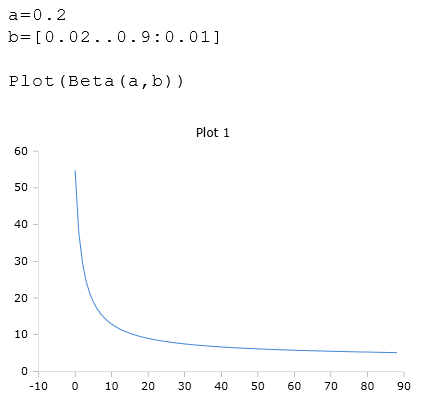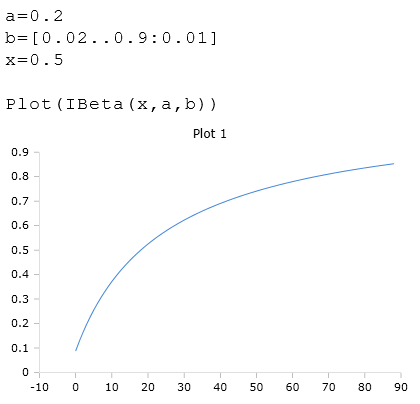# Beta Functions

Euler's beta functions

## Description of the beta function

The function $$Beta$$ (Euler's beta function), also Euler's integral type 1 is a mathematical function of two complex numbers. Their definition is:

$$\displaystyle Beta(a,b) = \int_0^1 t^{a-1}(1-t)^{b-1} dt$$

$$Beta$$ calculates the beta function for the arguments $$a$$ and $$b$$. The arguments must be real and 0 or positive.

You can also use a list in one of the parameters.

Beta (a, b)

### Beispiel## IBeta Function

The function $$IBeta$$ calculates the incomplete regulated beta function for the arguments $$a$$ and $$b$$. The arguments must be real and 0 or positive.

One of the arguments can also be a list of real numbers.

The parameter $$x$$ is the upper bound of the integral in the range: $$1 >= x >= 0$$

IBeta(x, a, b)

### Example